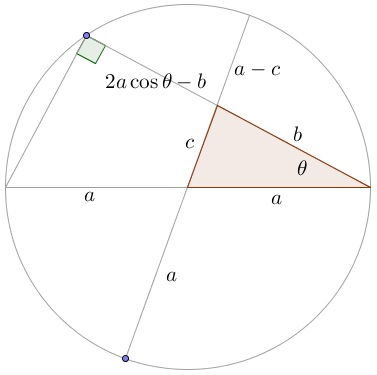# The Cosine Law: PWW by S. Kung

This is a proof by Sidney H. Kung:By the Intersecting Chords Theorem,

$(a+c)(a-c)=(2a\cos\theta-b)b,$

i.e., $a^2-c^2=2ab\cos\theta -b^2.$

### References

1. R. B. Nelson, Proofs Without Words, MAA, 1993, p. 32